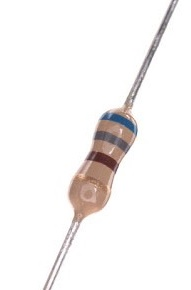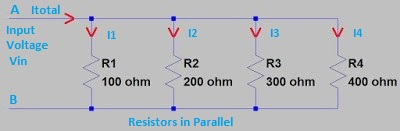# Parallel Resistor Calculator

Parallel Resistor Calculator will help you to fast calculate equivalent resistance value of 10 resistors connected in parallel.

Please insert the resistance values of the resistors in empty cells to calculate resistance value of parallel resistors.

R1
R2
R3
R4
R5
R6
R7
R8
R9
R10

Calculated Parallel Resistance

Required Decimal Value

An excel sheet also you can download by clicking below symbol, which you can use any time by saving it in your laptop or mobile.

Below are the details about resistor, resistor working, resistors in series and resistors in parallel.

### Resistor

A resistor is a passive component. Work of Resistor is to oppose the movement of current across it.Resistor

Resistor also adjusts signal stages, dividing the voltage in voltage divider circuit, to bias the component like transistors, resistive loads for the circuits, etc.

### Resistor Symbol

Below image shows symbol of both fixed type resistors.Resistor Symbol

### Resistor Working

Below points presents details for resistor working;

A resistor opposes current because flowing electrons strike with the atoms in the resistor and make them to vibrate.

This results in electrical energy to change in heat energy. Resistance “R” is the measure of the quantity by which a resistor opposes the flow of charge.

### Resistor works as per Ohm's law

As we know Ohm’s law is; V = I / R. This means basic principle of working of resistor or in the other words behaviour of the resistor working is based on the Ohm’s law.

Let’s clarify this; as per Ohm's law the voltage (V) passing through a resistor is proportional to the current (I), where the constant of proportionality is the resistance (R) i.e. the electrical resistance of the conductor is constant.

We can say that, with the extra added voltage applied to a resistor the extra current flows through it.

Let’s clear it with an example; If a 200ohm resistor is connected across the positive and negative terminals of a 12V dc power supply, then a current of 12 / 200 = 0.06A will flow through that resistor.

### Parallel and Series resistors

By joining resistors in series or in parallel we can very well control the current. Let see it in detail;

Resistors in series

Resistors in “Series” stats that, the resistors are connected together or organised in daisy form i.e. in a single line. Whole current passing through the first resistor must also passes through the second resistor and then it passes through third and so on, because there’s no other path for the flow of current.

Schematic shown below, shows that the resistors R1, R2, R3 and R4 all are connected in series, in between A point and B point and a common current (I) passing through all of them;

Hence, the total current that is passing through all these resistors in series will be the similar or same at any point.

IR4 = IR3 = IR2 = IR1 = Itotal

Hence, the total resistance here is the addition of all the individual resistance of the resistors connected in series.

R1 + R2 + R3 + R4 = Rtotal = 100 ohm + 200 ohm + 300 ohm + 400 ohm = 1k ohm

So, finally the total voltage across each resistor will be not same and can be calculated by the Ohm’s law. So, total voltage through the resistors is equal to the sum of the potential differences across each of the resistor.

Vtotal = VAB = VR1 + VR2 + VR3 + VR4 = 1V + 2V + 3V + 4V = 10V

Resistors in Parallel

Resistors in “Parallel” stats that, both the terminals of resistor or resistors are independently or individually connected to each terminal of the other resistor or resistors.

Schematic shown below shows that the resistors R1, R2, R3 and R4 are connected in the parallel, in between A point and B point and uncommon current (I) is passing through them;Resistors in Parallel

Hence, here in any branch the current passing across the resistors will be not same.

I1 + I2 + I3 + I4 = Itotal.

If Vin = 10V then;

Itotal = 0.1A + 0.05A + 0.03A + 0.025 = 0.205A

Here the addition of reciprocal (1/R) value of the individual resistances gives the total resistance. We can say that, the inverse of the equal resistance of two or more resistors joined or connected in the parallel is the addition of the inverses of each single resistance.

(1/R1) + (1/R2) + (1/R3) + (1/R4) = 1/Rtotal

= (1/100 ohm) + (1/200 ohm) + (1/300 ohm) + (1/400 ohm)

= 0.01 + 0.005 + 0.003 + 0.0025

= 0.0205 ohm

Rtotal = 1 / 0.0205 = 48.78 ohm

Hence, here the voltage across each of the resistor will be same, i.e. the voltage drop through all of the resistors in a parallel resistive circuit is similar.

Vin = VR1 = VR2 = VR3 = VR4 = 10V

The parallel resistor connection has the same voltage through it, the resistances might be dissimilar, and thus the current passing across each resistor will be different as per Ohms Law.

### Conclusion

In electronic circuits resistors are mostly used. All types of circuits it is present. Correct resistance value selection is mandatory in circuit design otherwise excessive current will damage the circuit. The series and parallel resistance calculator will help you to select right resistance.

For calculations of Resistor Wattage please visit, Resistor Wattage Calculator.

For calculations of Resistor for LED please visit, Resistor for LED Calculator.